# Triangulation

(diff) ← Older revision | Latest revision (diff) | Newer revision → (diff)
A triangulation of a polyhedron, or rectilinear triangulation, is a representation of the polyhedron (cf. Polyhedron, abstract) as the space of a geometric simplicial complex, that is, a decomposition of it into closed simplices such that any two simplices either do not intersect or intersect along a common face. Rectilinear triangulations of polyhedra serve as the main tool for studying them. Any polyhedron has a triangulation and any two triangulations of it have a common subdivision.
The closed starof a simplexin a triangulationis the union of the simplices ofcontaining. There is a representation of the closed star of a simplex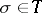as the union (or join, cf. Union of sets) ofand its link:. In particular, the star of a vertex is a cone over its link. If a simplexis represented as the join of two of its facesand, then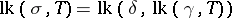. The link of a simplex does not depend on: Ifis a simplex in rectilinear triangulations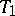,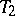of the same polyhedron, then the polyhedra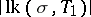and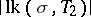are PL-homeomorphic. The open star of a simplexis defined as the union of the interiors of those simplices ofcontainingas a face. The open stars of the vertices of a triangulation of a polyhedronform an open covering of. The nerve of this covering (cf. Nerve of a family of sets) is simplicially isomorphic to the triangulation. Two triangulationsandof polyhedraand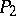are combinatorially equivalent if certain subdivisions of them are simplicially isomorphic. In order that two triangulationsandbe combinatorially equivalent it is necessary and sufficient that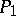andbe PL-homeomorphic. A triangulation of a manifold is said to be combinatorial if the star of any of its vertices is combinatorially equivalent to a simplex. In this case the star of any simplex of the triangulation is also combinatorially equivalent to a simplex.
Ifis a closed subpolyhedron of a polyhedron, then any triangulationofcan be extended to some triangulationof. In this case one says that the pair of geometric simplicial complexes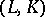triangulates the pair. A triangulation of the direct productof two simplices,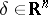can be constructed as follows. The vertices of the triangulation are the points,, where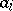are the vertices ofand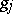are the vertices of. The vertices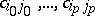, where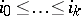, span a-dimensional simplex if and only if none of these coincide and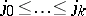. A triangulation of the direct product of two simplicial complexes with ordered vertices can be carried out in the same way.
A triangulation of a topological space, or curvilinear triangulation, is a pair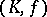, whereis a geometric simplicial complex and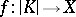is a homeomorphism. Two triangulationsandof a spacecoincide ifis a simplicial isomorphism. Ifis a simplex of a complexandis a triangulation of, then the space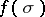endowed with the homeomorphism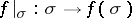is called a topological simplex. The star and the link of a topological simplex of a triangulated topological space are defined in the same way as in the case of rectilinear triangulations. If a point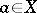is a vertex of triangulationsandof, then its links in these triangulations are homotopy equivalent.